Spam classifier example, error analysis, skewed data, precision, recall and large data sets.

## 1. Building a Spam Classifier

I would like to give full credits to the respective authors as these are my personal python notebooks taken from deep learning courses from Andrew Ng, Data School and Udemy :) This is a simple python notebook hosted generously through Github Pages that is on my main personal notes repository on https://github.com/ritchieng/ritchieng.github.io. They are meant for my personal review but I have open-source my repository of personal notes as a lot of people found it useful.

### 1a. Prioritizing

• Let’s say you want to build a spam classifier
• How do you implement supervised learning?
• We can create the following
• x = features of email
• Choose 100 words indicative of spam or not spam
• In practice is to look through training set and choose most frequently occurring n words (10 000 to 50 000)
• y = spam (1) or non-spam (0)
• Example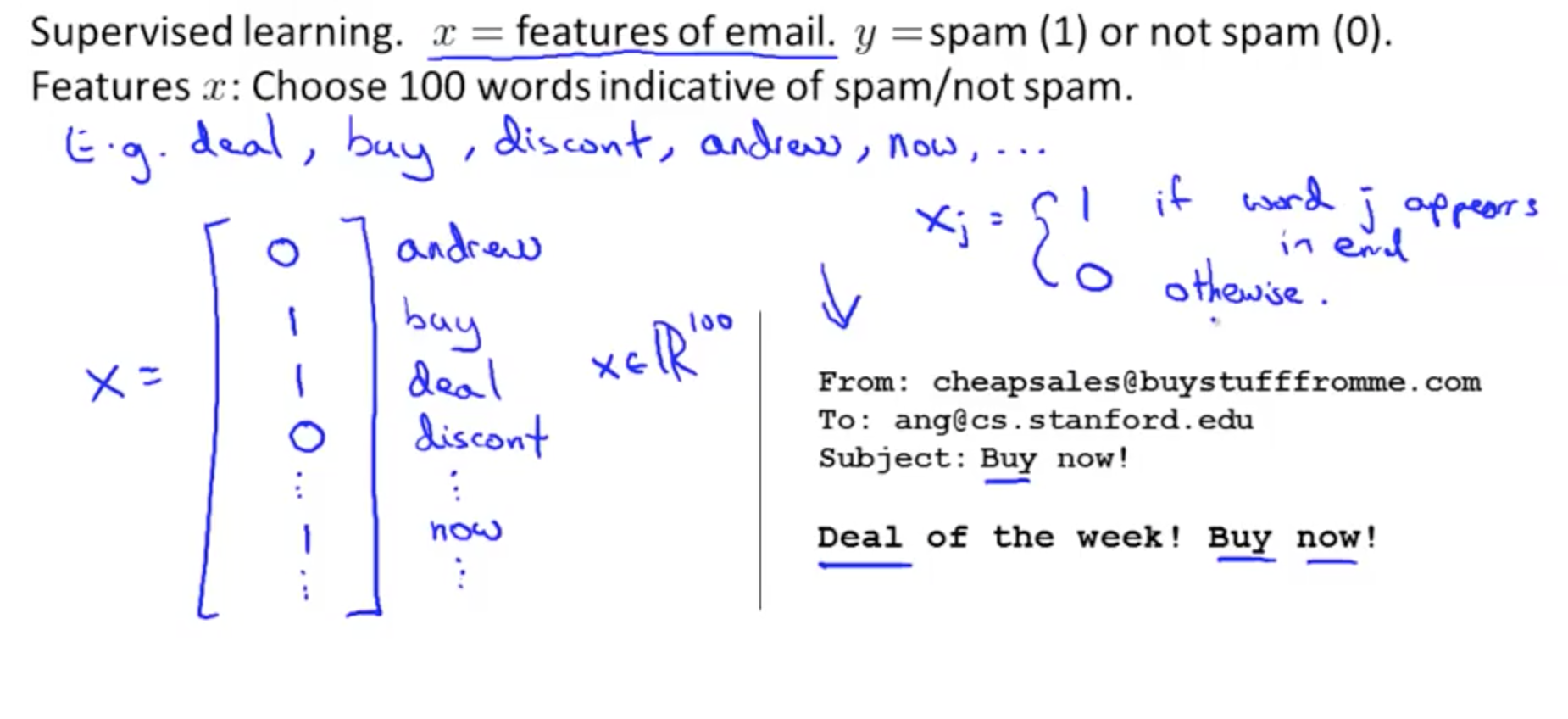• How do you spend your time to have a low error?
• Collect lots of data
• Develop sophisticated features based on email routing information (from email header)
• Develop sophisticated features for message body
• Should ‘discount’ and ‘discounts’ be treated as the same word?
• How about ‘deal’ and ‘Dealer’?
• Punctuation?
• Develop sophisticated algorithm to detect misspellings
• med1cine
• w4tches
• m0rtage
• Don’t base on your gut feeling!

### 1b. Error Analysis

• Recommended Approach
• Start with a simple algorithm that you can quickly implement and test it on your cross validation data
• Plot learning curves to decide if more data, more features, etc. are likely to help
• Error analysis:
• Manually examine the examples (in cross validation set) that your algorithm made errors on
• See if you spot any systematic trend in what type of examples it is making errors on
• Don’t base anything off your gut feeling!
• Error Analysis Example
• m_cv = 500
• number of cross-validation examples
• Algorithm misclassified 100 emails
• Manually examine the 100 errors and categorize them base don
• What type of email they are
• Pharma: 12
• Replica: 4
• Phishing: 53
• Other: 31
• What cues (features) you think would have helped the algorithms classify them correctly
• Deliberate misspellings: 5
• m0rtgage
• med1cine
• This indicates how there are a small number here
• Unusual email routing: 16
• Unusual punctuation: 32
• This might be worthwhile to spend time to develop sophisticated features
• This is the reason why we should do a quick and dirty implementation to discover errors and identify areas to focus on
• Importance of numerical evaluation
• Should discount, discounts, discounted, discounting etc. be treated as the same word?
• You can use a “stemming” software, “Porter Stemmer”
• This would allow you to treat all those variations as the same work
• Software may mistake universe and university as the same word
• Error analysis may not be helpful for deciding if this is likely to improve performance
• The only way is to try it
• We need a numerical solution (cross validation error)of algorithm’s performance with and without stemming
• Without stemming: 5%
• With stemming: 3%
• This implies that it may be useful to implement stemming
• Distinguish between upper and lower case: 3.2%
• Why is the recommended approach to perform error analysis using the cross validation data instead of the test data?
• If we develop new features by examining the test set, then we may end up choosing features that work well specifically for the test set, so Jtest(θ) is no longer a good estimate of how we generalize to new examples
• Do error analysis on cross validation set, do not use it on the test set!

## 2. Handling Skewed Data

### 2a. Error Metrics for Skewed Classes

• Consider a problem where you want to find out if someone has cancer
• y = 1, cancer
• y = 0, no cancer
• You train a logistic regression model, h0(x) and you find that you have 1% error on a test set
• 99% correct diagnosis
• But only 0.50% of patients have cancer
• This is a problem of skewed classes
• This code would have a 0.5% error, lower than your logistic regression model but it’s simply predicting based on 0.5% of patients who have cancer
  function y = predictCancer(x)
y = 0;
return

• Let’s say you have
• 99.2% accuracy
• 0.8% error
• If you improve your algorithm to become 99.5% accuracy
• 0.5% error
• It might be the case of just predicting whether you have cancer that would yield this error
• Precision/Recall
• By calculating precision/recall, we will have a better sense of how our algorithm is doing
• If y = 0
• Recall = 0
• This shows that the classifier is not good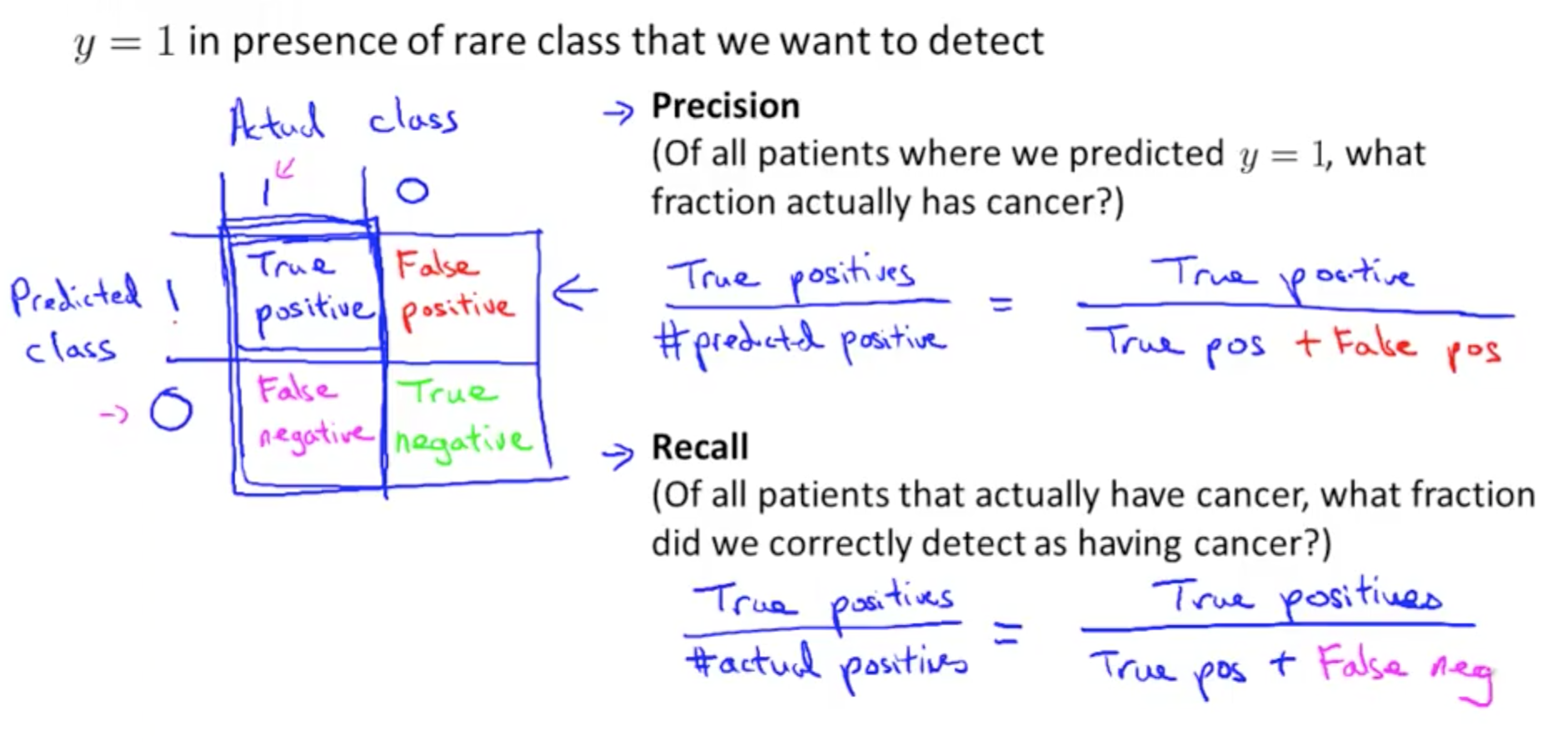### 2b. Trading off Precision and Recall

• If we want to avoid false positives
• We want to be more confident before predicting cancer (y = 1)
• We can increase the threshold of h0(x) from 0.5 to 0.7 or even 0.9
• Result
• False positives: decrease
• True positives: decrease
• Recall: decrease
• Precision: higher
• If we want to avoid false negatives
• We want to avoid missing too many cases of cancer
• We can decrease the threshold of h0(x) from 0.5 to 0.3
• Result
• False negatives: decrease
• True positive: increase
• Recall: higher
• Precision: lower
• Many different precision recall curve, but here is one example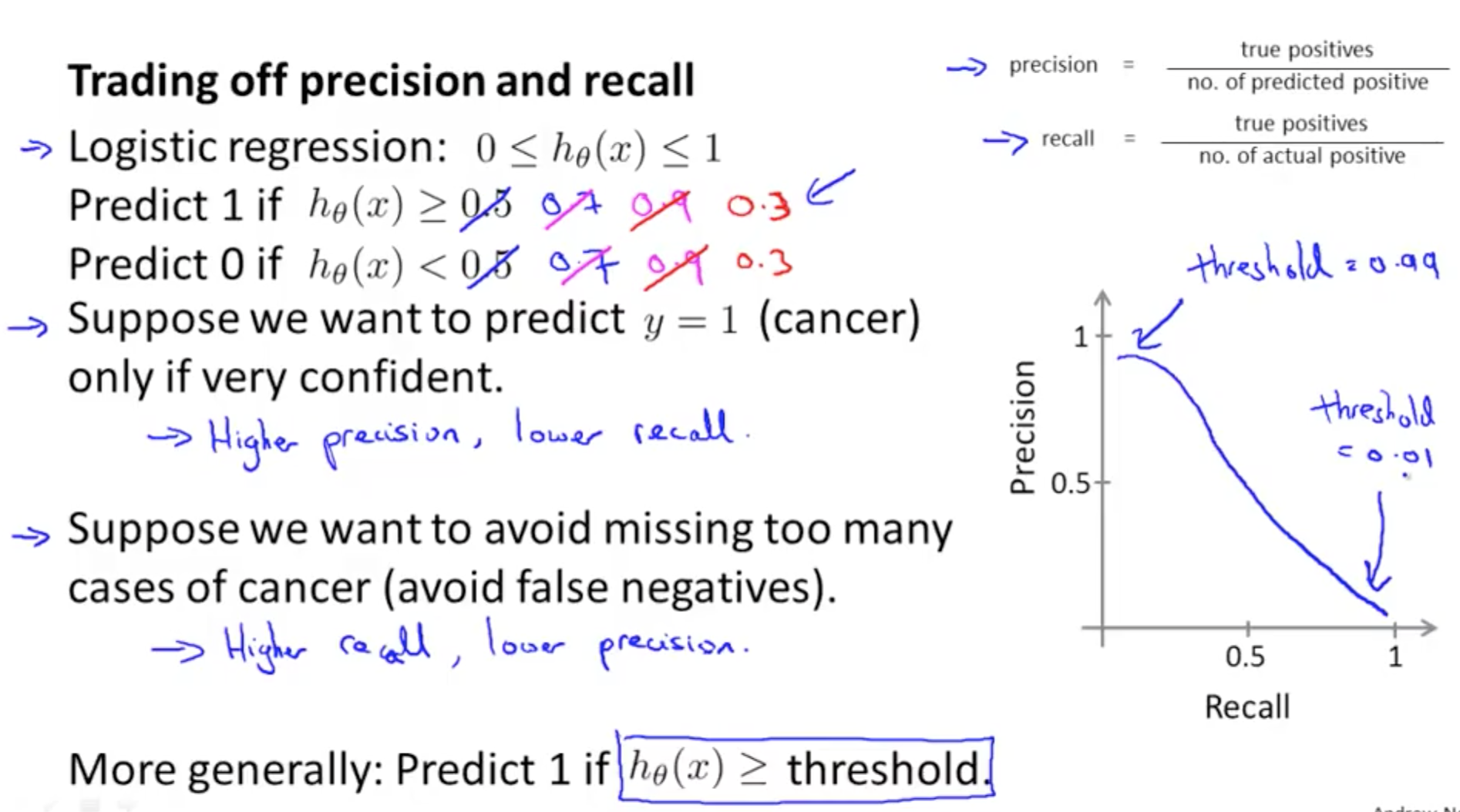• How do we compare precision/recall numbers? Which pair is best?
• We can use an average
• At the extremes, neither classifiers is good
• If we predict y = 1 all the time, it’s a useless classifier even though if it has a high recall
• But average is not good because the extreme scenarios may have a lower average than other combinations that may be better
• We should use the F score (F1 score)
• F1 Score = (2 * P * R) / (P + R)
• Remember to measure P and R on the cross-validation set and choose the threshold which maximizes the F-score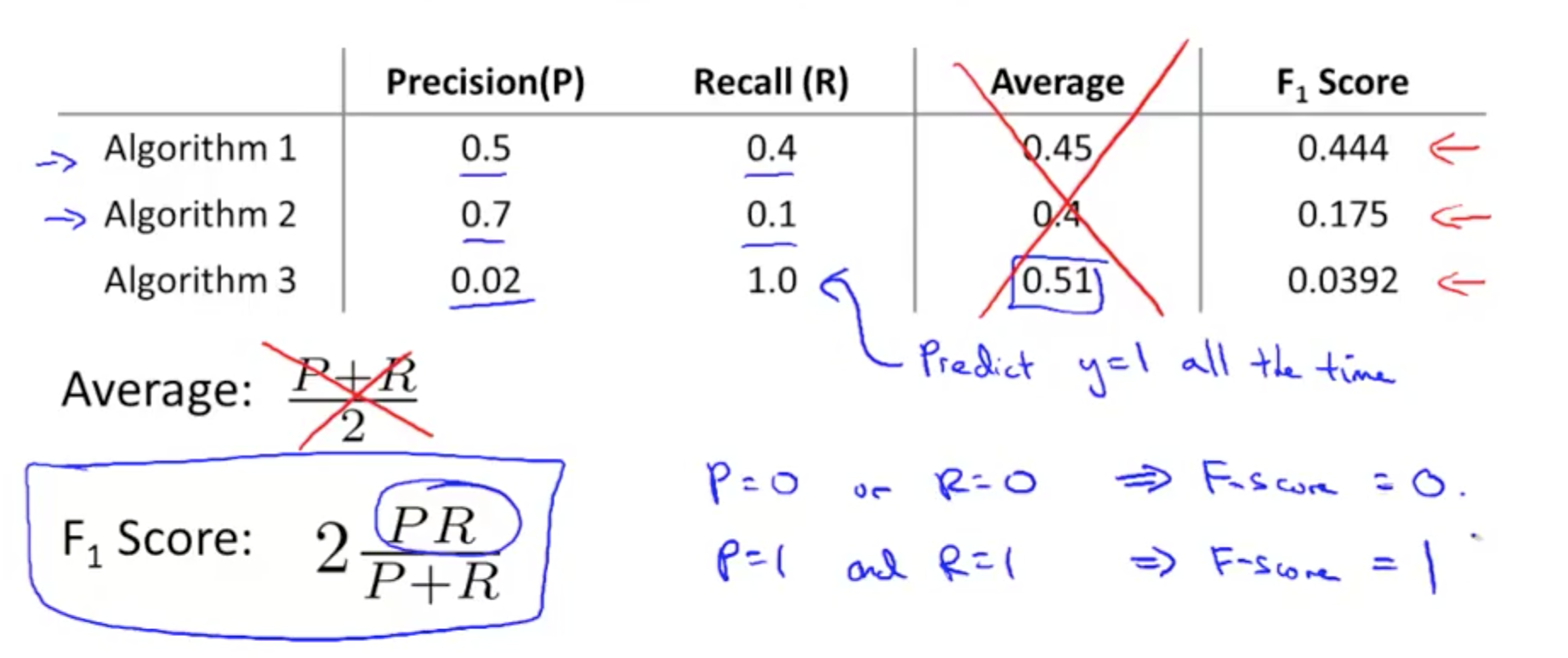## 3. Using Large Data Sets

• Under certain conditions, getting a lot of data and training a learning algorithm would result in very good performance
• Designing a high accuracy learning system
• Classify between confusable words
• to, two, too
• then, than
• For breakfast, I ate two eggs
• Algorithms
• Perceptron (logistic regression)
• Winnow (less popular)
• Memory-based (less popular)
• Naive Bayes (popular)
• Algorithms give roughly similar performance
• With larger training set, all algorithms’ precision increase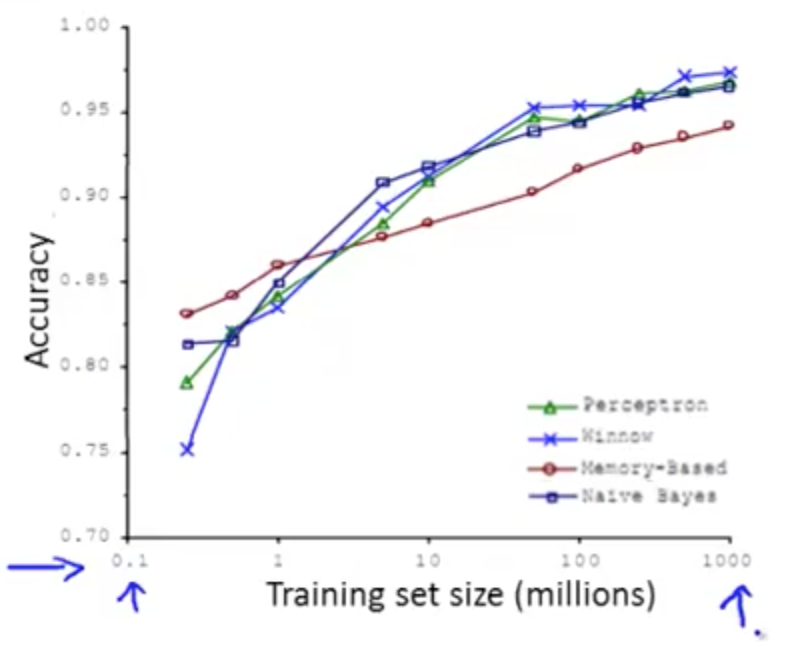• Often, it is not who has the best algorithm, but who has the most data
• Large data rationale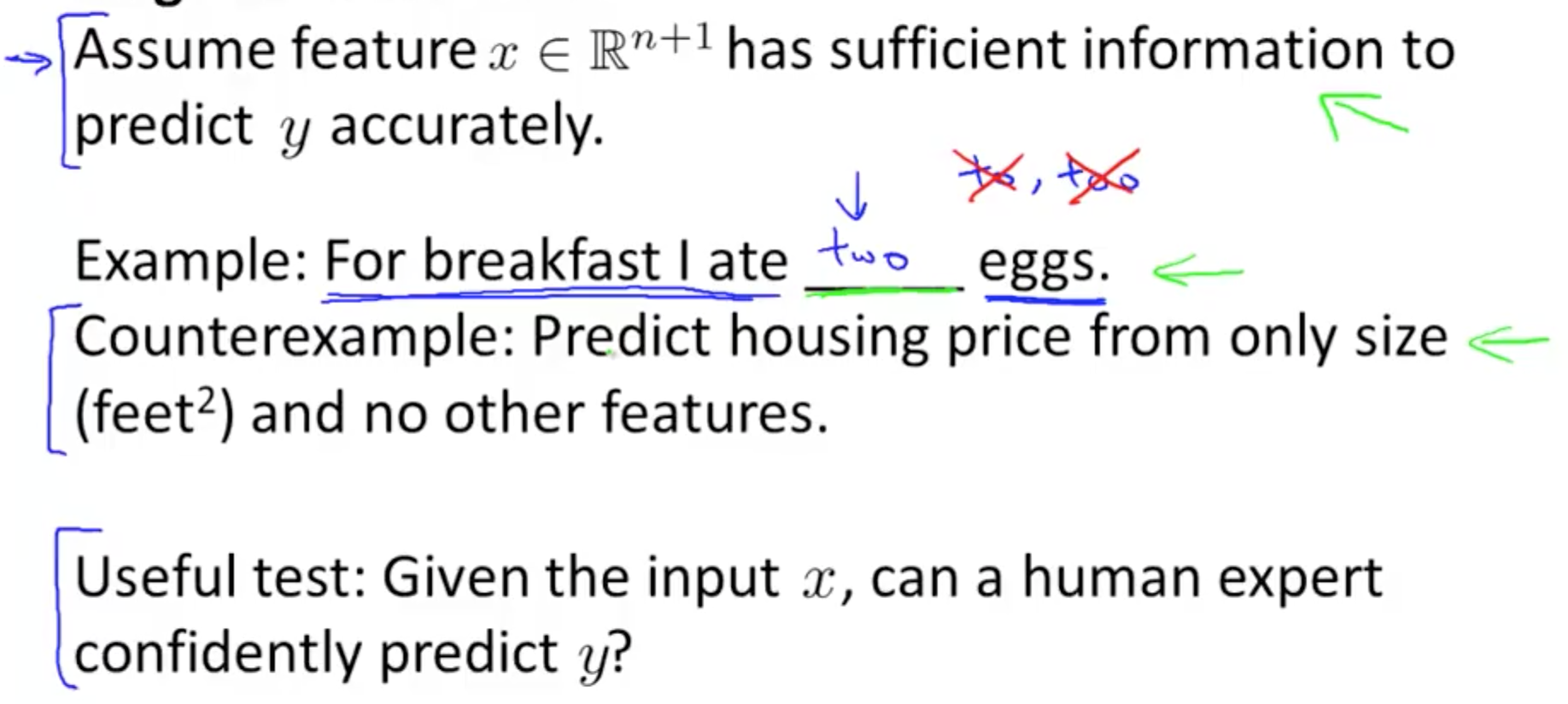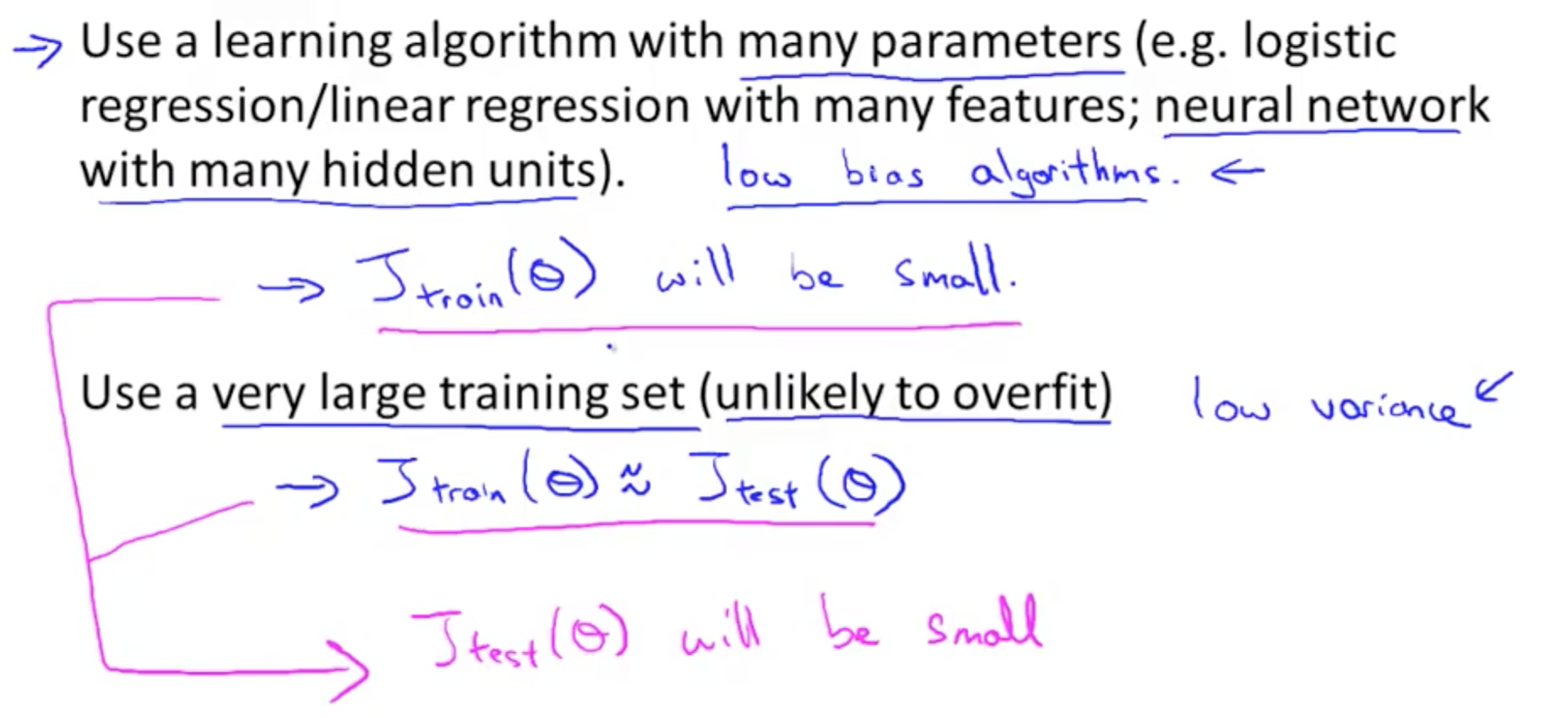• In sum
• Low bias: use complex algorithm
• Low variance: use large training set
Tags: